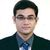# QlikView App Dev

Discussion Board for collaboration related to QlikView App Development.

Announcements
Skip the ticket, Chat with Qlik Support instead for instant assistance.
cancel
Showing results for
Did you mean:Anonymous
Not applicable

## Calculation in load script using master calendar dates

Dear all,

I have need some expert advise for my problem

I have created a master calendar in my project and also I have table which has 2 date fields.

Sample 2 rows from my data:

01/01/201631/12/201610001
01/02/201601/02/20175002

I have a monthenddate field in my master calendar which is basically last day of the each month.

My formula is : (monthenddate - Start Date) / (End Date - Start Date) * Premium

I would like to run this formula in load script for each contract for the each month end date and my I should reach a data set which has

12 different rows for each contract after running this formula like

Policy no, Monthenddate, Function Result

1                  31/01/2016          10

1                  28/02/2016           15

1                  31/03/2016            20

.......

1                   31/12/2016          100

Many thanks,

1 Solution

Accepted SolutionsMVP

Another alternative to Kaushik's solution

Table:

((MonthEndDate - [Start Date])/([End Date] - [Start Date])) * Premium as ResultField;

Date(Floor(MonthEnd([Start Date], IterNo()-1))) as MonthEndDate

While IterNo() <= Difference;

[End Date],

(Year([End Date]) * 12 + Month([End Date])) - (Year([Start Date]) * 12 + Month([Start Date])) + 1 as Difference,

ContractNo

FROM

(html, codepage is 1252, embedded labels, table is @1);4 RepliesHi,

Have a look at the attached Example.

Regards,

Kaushik Solanki

Regards,
KaushikMVP

Another alternative to Kaushik's solution

Table:

((MonthEndDate - [Start Date])/([End Date] - [Start Date])) * Premium as ResultField;

Date(Floor(MonthEnd([Start Date], IterNo()-1))) as MonthEndDate

While IterNo() <= Difference;

[End Date],

(Year([End Date]) * 12 + Month([End Date])) - (Year([Start Date]) * 12 + Month([Start Date])) + 1 as Difference,

ContractNo

FROM

(html, codepage is 1252, embedded labels, table is @1);Anonymous
Not applicable
Author

Dear kaushik.solanki‌ would you write your script in here since I have personal edition.

Thanks,

EEHere is the script.

DATA:

Load *,[Start Date]&'-'&[End Date] as Key;

LOAD Date(Date#([Start Date],'DD/MM/YYYY')) as [Start Date],

Date(Date#([End Date],'DD/MM/YYYY')) as [End Date],

ContractNo

FROM

(html, codepage is 1252, embedded labels, table is @1);

MINMAX:

Max([End Date]) as MAX

Resident DATA;

Let vMin = num(Peek('MIN',0,'MINMAX'));

Let vMax = num(Peek('MAX',0,'MINMAX'));

Temp:

Load Floor(MonthEnd(Date(\$(vMin) + RowNo() -1))) as MonthEnd

AutoGenerate 1

While Date(\$(vMin) + RowNo() -1) < Date(\$(vMax));

INTERVAL:

intervalmatch(MonthEnd)

Load [Start Date],[End Date] Resident DATA;

Left join(DATA)

Load distinct [Start Date]&'-'&[End Date] as Key,MonthEnd

Resident INTERVAL;

Drop table INTERVAL;

FINAL:

Load *,(MonthEnd - [Start Date]) / ([End Date] - [Start Date]) * Premium as Value Resident DATA;

Drop table DATA, MINMAX;

Regards,

Kaushik Solanki

Regards,
Kaushik